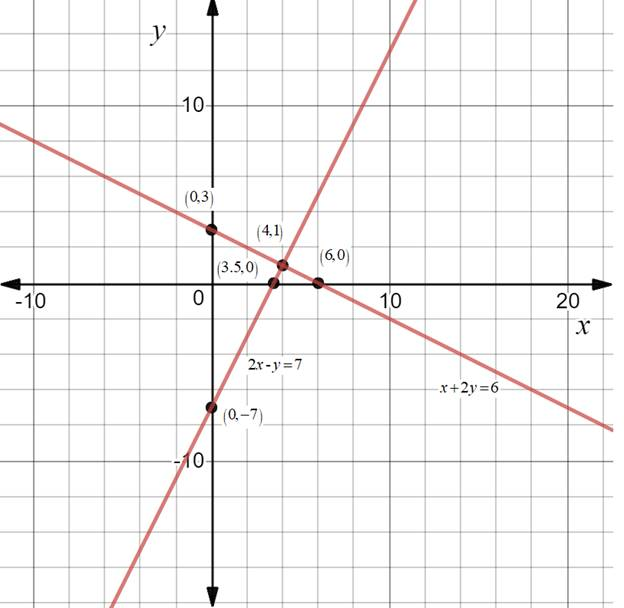Chapter 10.CT, Problem 18CT### Elementary Geometry for College St...

6th Edition
Daniel C. Alexander + 1 other
ISBN: 9781285195698

#### Solutions

Chapter
Section### Elementary Geometry for College St...

6th Edition
Daniel C. Alexander + 1 other
ISBN: 9781285195698
Textbook Problem
1 views

# Use the graphs provided to solve the system consisting of the equations x + 2 y = 6 and 2 x - y = 7 .                                         _To determine

To solve:

The system consisting of the equations x+2y=6 and 2x-y=7 using the given graph.Explanation

It is observed from the graph that the point of intersection is (4,1)

### Still sussing out bartleby?

Check out a sample textbook solution.

See a sample solution

#### The Solution to Your Study Problems

Bartleby provides explanations to thousands of textbook problems written by our experts, many with advanced degrees!

Get Started

#### Subtract: (2)(0)

Elementary Technical Mathematics

#### What is the value of (X + 1) for the following scores: 0, 1, 4, 2? a. 8 b. 9 c. 11 d. 16

Essentials of Statistics for The Behavioral Sciences (MindTap Course List)

#### limx(12)x2= a) 0 b) 1 c) d) does not exist

Study Guide for Stewart's Single Variable Calculus: Early Transcendentals, 8th